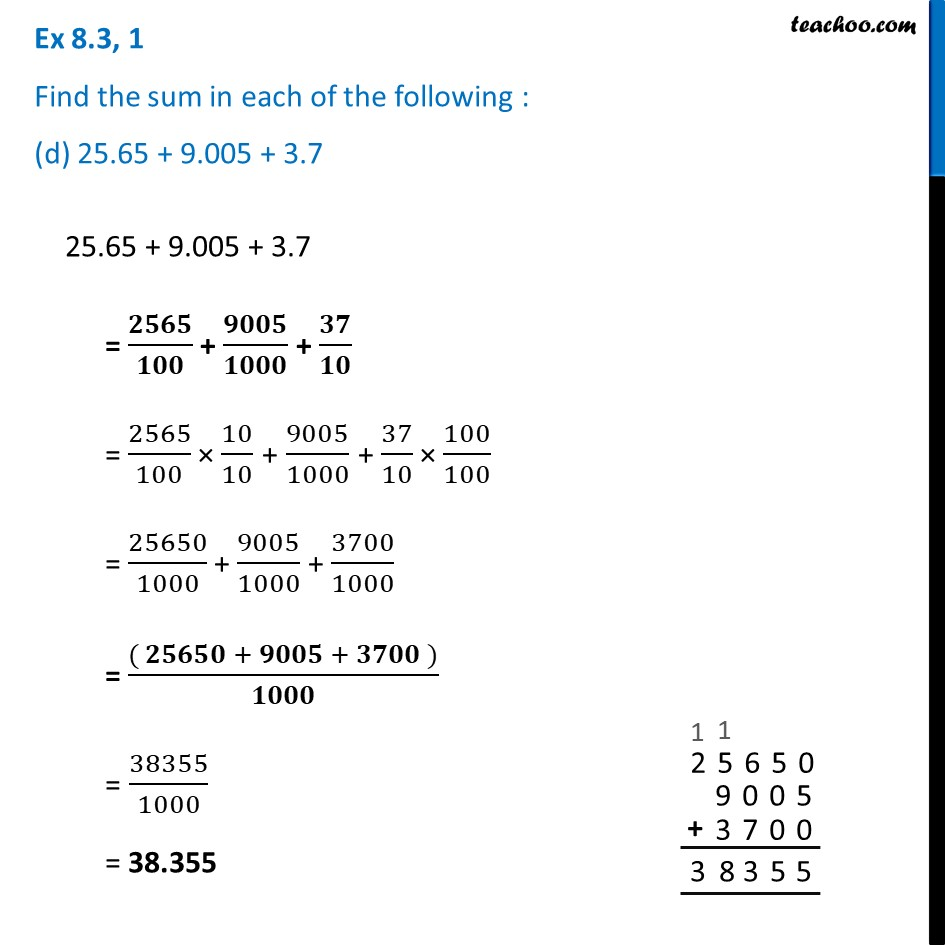Ex 8.3

Chapter 8 Class 6 Decimals
Serial order wiseLearn in your speed, with individual attention - Teachoo Maths 1-on-1 Class

### Transcript

Ex 8.3, 1 Find the sum in each of the following : (d) 25.65 + 9.005 + 3.725.65 + 9.005 + 3.7 = 𝟐𝟓𝟔𝟓/𝟏𝟎𝟎 + 𝟗𝟎𝟎𝟓/𝟏𝟎𝟎𝟎 + 𝟑𝟕/𝟏𝟎 = 2565/100 × 10/10 + 9005/1000 + 37/10 × 100/100 = 25650/1000 + 9005/1000 + 3700/1000 = (( 𝟐𝟓𝟔𝟓𝟎 + 𝟗𝟎𝟎𝟓 + 𝟑𝟕𝟎𝟎 ))/𝟏𝟎𝟎𝟎 = 38355/1000 = 38.355